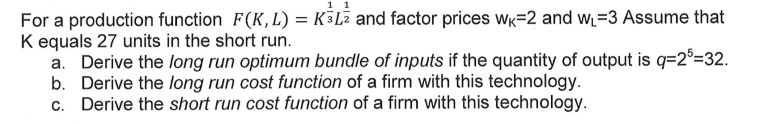# For a production function F(KL) = K-L2 and factor prices wK-2 and WL-3 Assume that K equals 27 un...For a production function F(KL) = K-L2 and factor prices wK-2 and WL-3 Assume that K equals 27 units in the short run a. Derive the long run optimum bundle of inputs if the quantity of output is q-25-32. b. Derive the long run cost function of a firm with this technology. c. Derive the short run cost function of a firm with this technology.

q = F(K, L) = K1/3L1/2

(a)

In long run, cost is minimized when MPL/MPK = wL/wK = 3/2

MPL =q/L = (1/2) x K1/3 / L1/2

MPK =q/K = (1/3) x L1/2 / K2/3

MPL/MPK = [(1/2) x K1/3 / L1/2] / [(1/3) x L1/2 / K2/3] = (3/2) x (K/L) = 3.2

K/L = 1

L = K

Substituting in production function with q = 32,

K1/3L1/2 = 32

L1/3L1/2 = 32

L5/6 = 32

Taking (6/5)th root,

L = 64

K = 64

(b)

MPL/MPK = K/L = 3/2

K = 3L/2

Substituting in generalized production function,

(3L/2)1/3L1/2 = q

(3/2)1/3L1/3L1/2 = q

1.14 x L5/6 = q

L5/6 = q / 1.14 = 0.88q

Taking (6/5)th root,

L = (0.88)6/5q6/5 = 0.85 x q6/5

K = (3/2) x L = 1.5 x 0.85 x q6/5 = 1.28 x q6/5

(c)

In short run, K = 27.

(27)1/3L1/2 = q

3L1/3L1/2 = q

3 x L5/6 = q

L5/6 = q / 3 = 0.33q

Taking (6/5)th root,

L = (0.33)6/5q6/5 = 0.27 x q6/5

K = 27

Total cost (TC) = L x wL + K x wK = 3L + 2K = 3 x (0.27 x q6/5) + 2 x 27

TC = 0.81 x q6/5 + 54

##### Add Answer of: For a production function F(KL) = K-L2 and factor prices wK-2 and WL-3 Assume that K equals 27 un...
More Homework Help Questions Additional questions in this topic.

• #### . Suppose the production function of a firm is given by q = L1/4K2/4. The prices of labor and cap...

Need Online Homework Help?